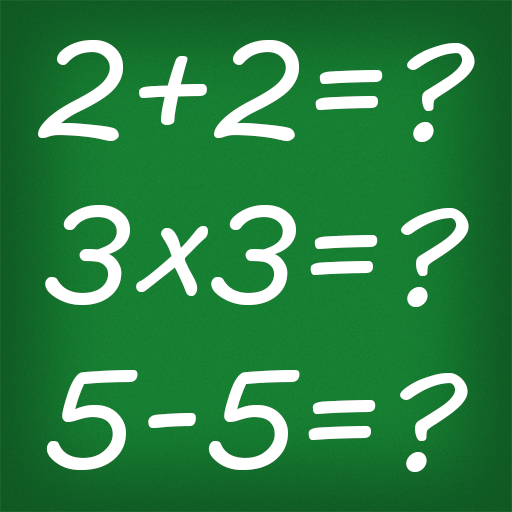# Math Games on PC/Mac

Author: Ocosys

Score: 3.9

OS: Windows, OS X, 4.2 +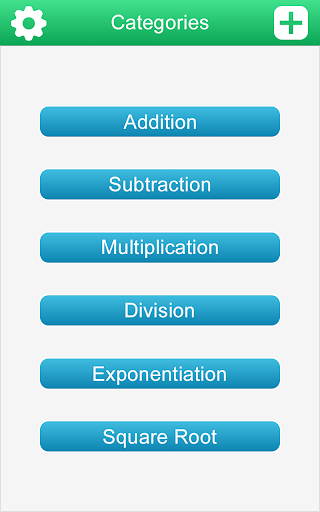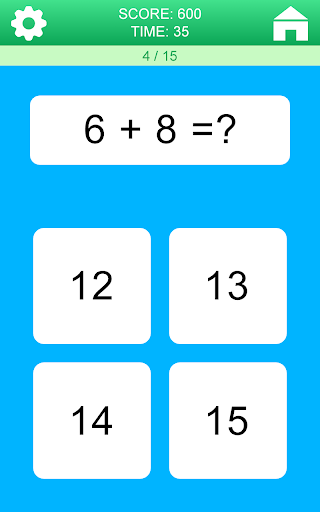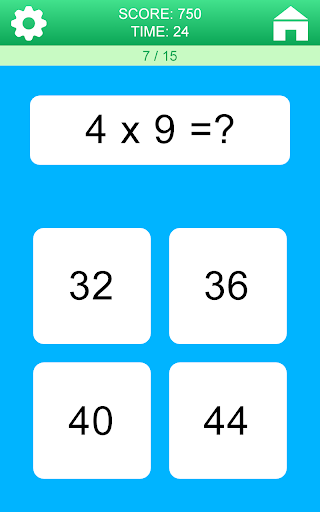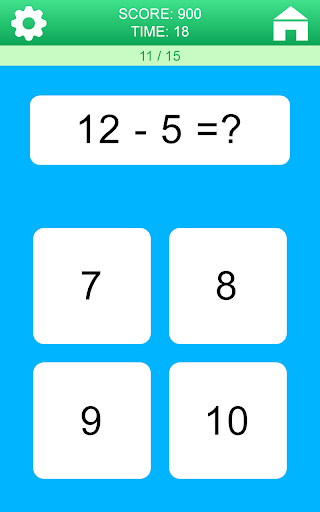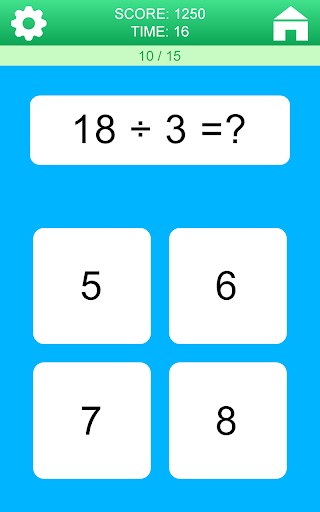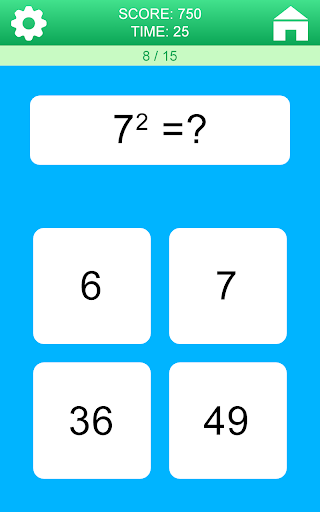Math Games application is designed for those who want to improve themselves in basic math operations.

You can improve your mathematical thinking skills by solving math questions in the app.

Math exercises are more fun with this free math game.

Our math game includes infinite numbers of math quizzes.

Game categories: Addition, subtraction, multiplication, division, exponentiation, square root